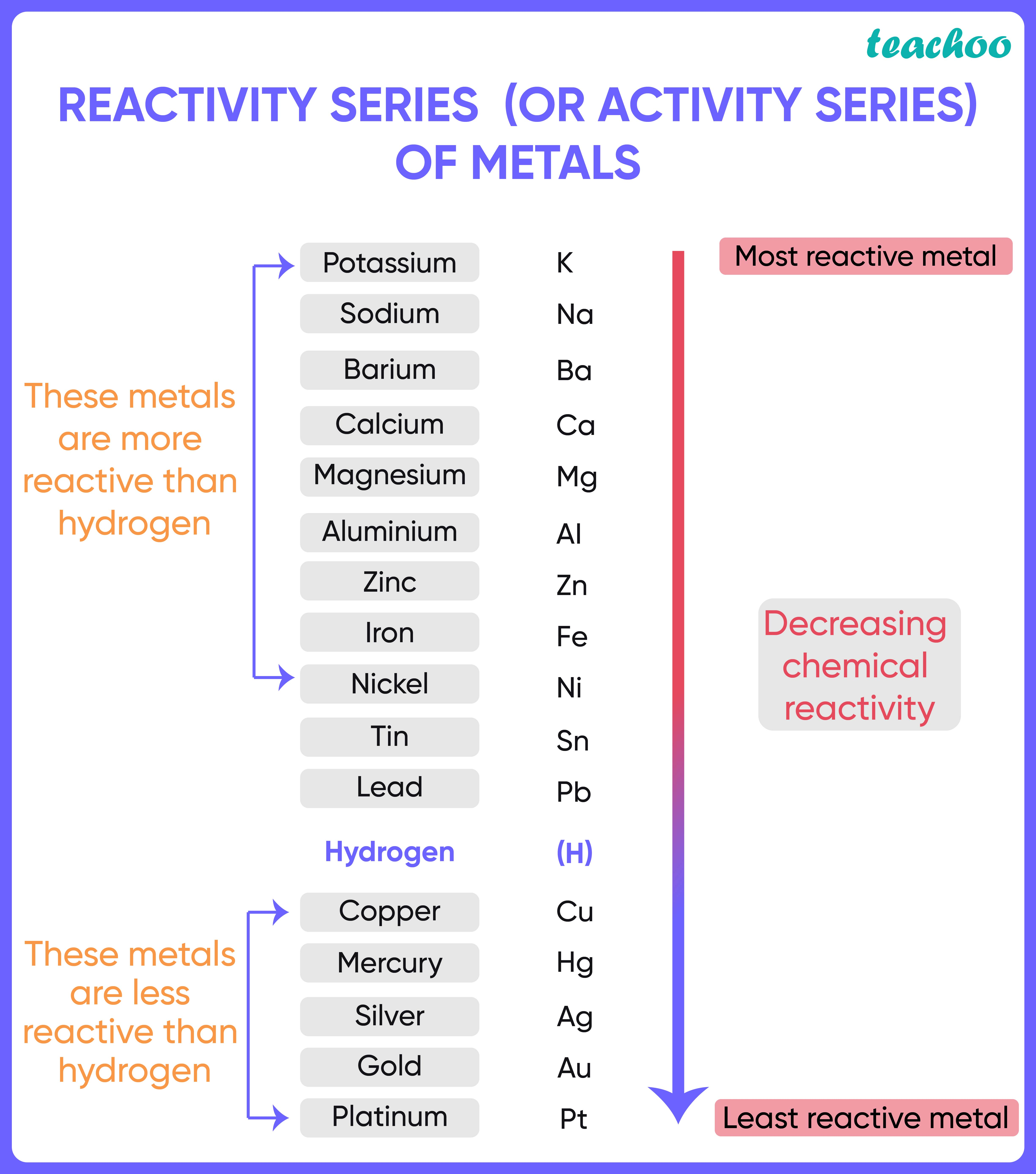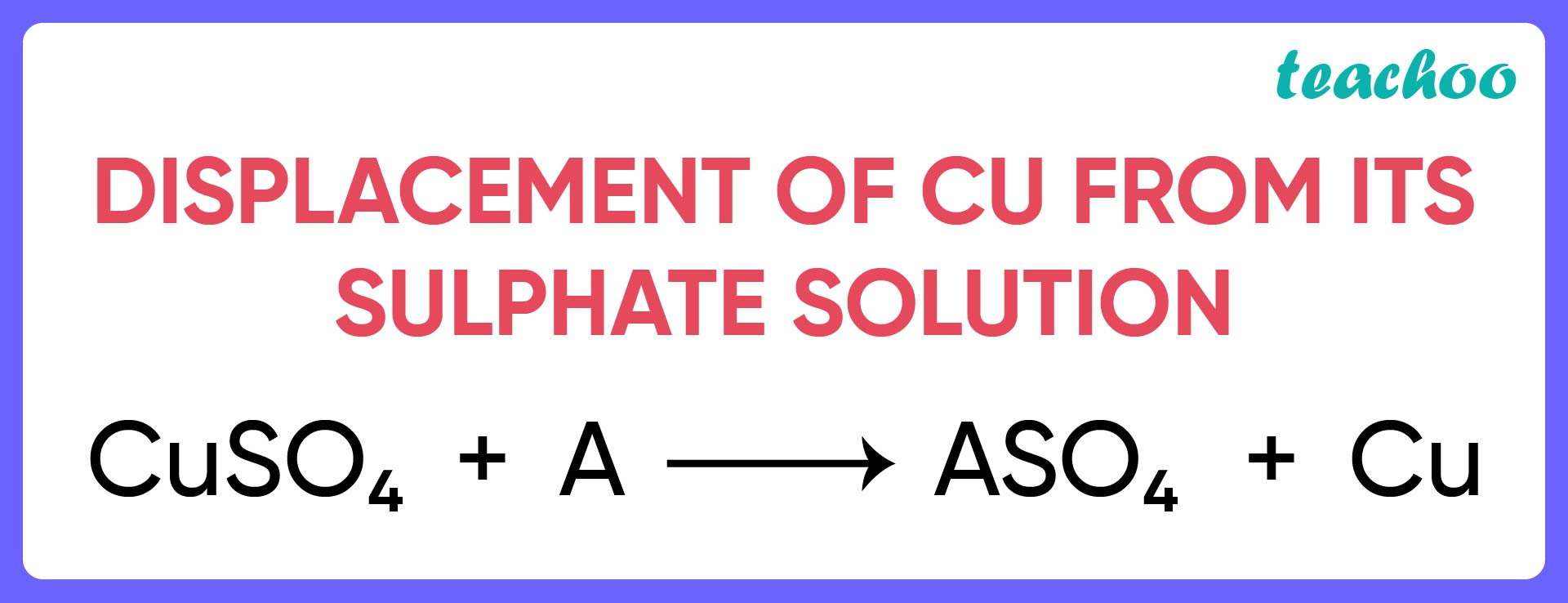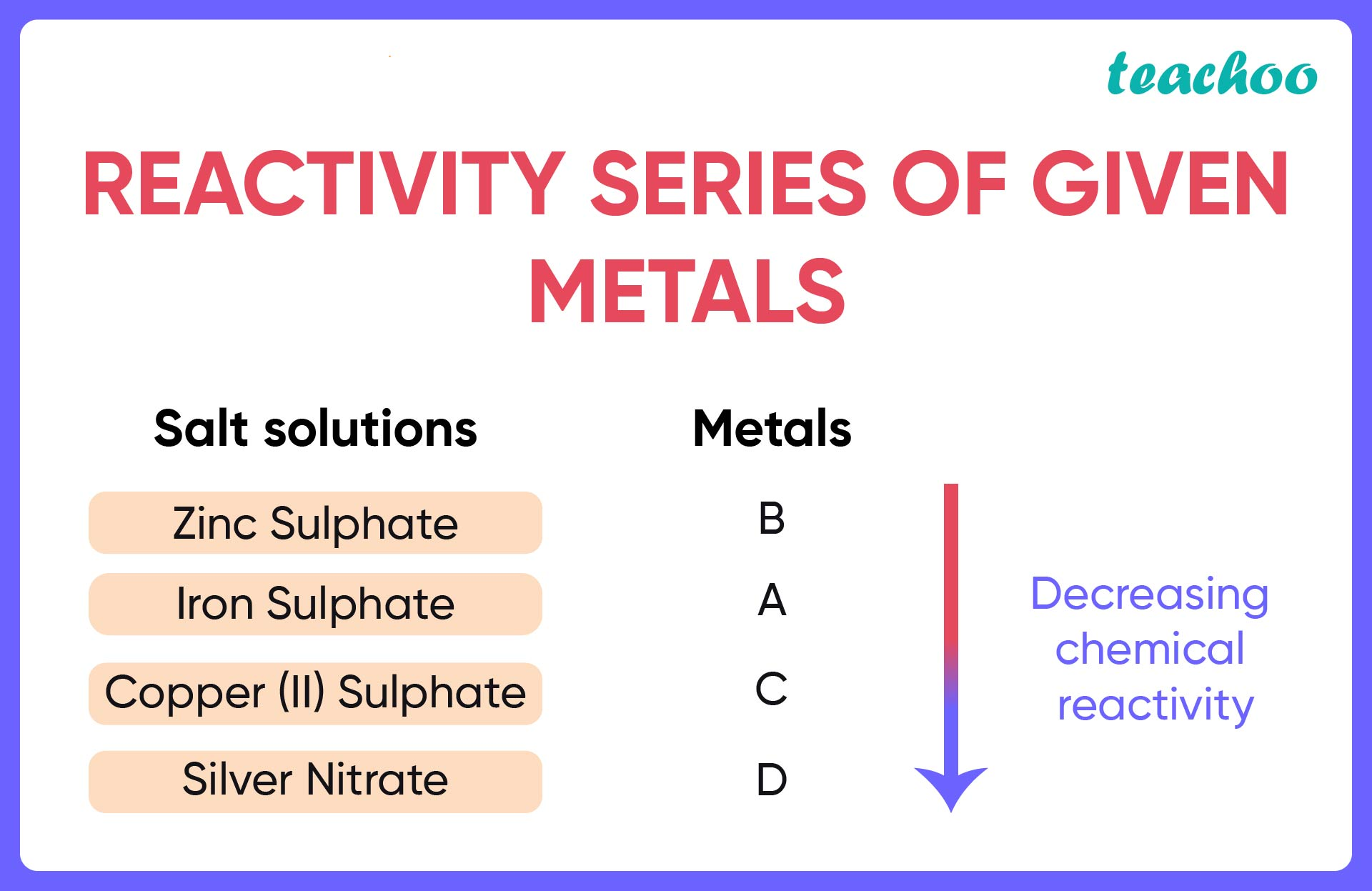Case Based Questions (MCQ)

Class 10
Chapter 3 Class 10 - Metals and Non-Metals

## Study the given table and answer any four questions from (a) to (e):  A student took the samples of four metals A, B, C and D and added the following solutions one by one. The results  obtained have been tabulated as follows:

 Metal Iron (II) Sulphate Copper (II) Sulphate Zinc Sulphate Silver Nitrate A No reaction Displacement - - B Displacement - No reaction - C No reaction No reaction No reaction Displacement D No reaction No reaction No reaction No reaction

### (iv) D

• According to the reactivity series , among the 4 salts given, Zinc Sulphate is the most reactive
• Since no metals react with it, we consider the next most reactive solution, Iron (II) Sulphate .
• Since only B is able to displace Iron from Iron (II) Sulphate, it is the most reactive among A,B,C,D

###googletag.cmd.push(function() { googletag.display('div-gpt-ad-1669298377854-0'); });

So, the correct answer is (i) B

### (iv) None of the above

From the given table, it is seen that

• A is able to displace Cu from Copper (II) Sulphate.
• Since B is more reactive than A , it will also be able to displace Cu.So, the correct answer is (iii) Both A and B

### (iv) B > A > D > C

Let us go in order of most reactive to least reactive salt solutions.

• Zinc solution is the most reactive salt solution here. But no metal reacts with it
• B is the most reactive element as it is able to displace Iron from its salt solution .
• A is able to displace copper from its salt solution.
• C is able to displace Silver from its salt solution.
• D is the least reactive element, since it does not react at all .So, the correct option is (i) B > A > C > D

### (iv) none of the above

The reaction of a reactive metal with dil HCl is as follows:

2M + 2dil HCl→ 2MCl+ H 2

Where M is any reactive metal.

So, the correct option is (iv) Hydrogen

### (iv) Most reactive: B; Least reactive: A

• A is able to displace B from its salt solution BX. So, A is more reactive than B
• C is able to displace A from its salt solution AY. So, C is more reactive than A and therefore, also more reactive than B

Hence, C is most reactive and B is least reactive

So, the correct option is (i) Most reactive: C; Least reactive: B

Learn in your speed, with individual attention - Teachoo Maths 1-on-1 Class